﻿ 多层高温作业服装模型的研究

多层高温作业服装模型的研究Research on Multi-Layer High Temperature Work Clothing Model

Abstract: The temperature difference between objects in an object or system is a necessary condition for generating heat conduction and the heat transfer rate (called heat conduction rate) caused by the heat conduction method is determined by the temperature distribution inside the object. The mechanism of heat conduction is more complicated. In 1822, French mathematician Fourier refined the heat conduction data and practical experience, and summarized the heat conduction law as Fourier’s law. For a uniform medium of a certain thickness, if there is a temperature difference between the two sides, the heat per unit area from the side with a high temperature to the side with a low temperature per unit time is proportional to the temperature difference between the two sides, and is inversely proportional to the thickness of the homogeneous. Specific heat capacity is the amount of heat absorbed or released by a unit mass object when it changes its unit temperature. After the problem analysis, we built a multi-layer protective clothing model and analyzed and solved each layer by differential equation method. For the first problem, heat conduction per unit area per unit time can be obtained, and the heat obtained per layer per unit time is the heat obtained from the previous layer minus the heat transferred to the next layer. Bringing the data of Annex I, using Mathematica to find the numerical solution of the differential equation in 5400 seconds, obtaining the function of temperature with respect to time, making an image, and the image generated by the calculated value is basically consistent with the image generated by the data provided in Annex II. After data review, heat transfer also includes the invisible effects of various parameters. Radiation heat transfer has the greatest influence on the parameters. There-fore, in the optimization of the model, we mainly optimize the radiant heat parameters based on the multi-layer high-temperature workwear model established by ourselves.

1. 引言

2. 问题重述

2.1. 背景介绍

2.2. 提出问题

2.3. 研究的意义

3. 问题分析

3.1. 问题一的分析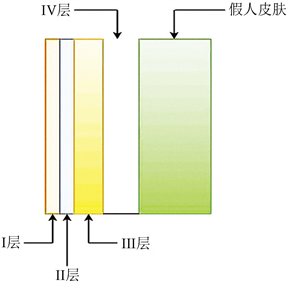Figure 1. Multi-layer high temperature work clothes concept map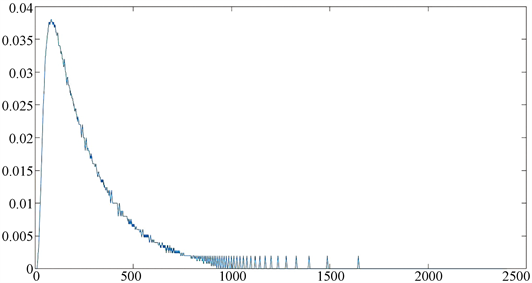Figure 2. Differential and softening

3.2. 问题二的分析

3.3. 问题三的分析

4. 模型建立、求解与检验

4.1. 问题一的分析、求解与检验

$\left\{\begin{array}{l}\frac{\text{d}{T}_{4}}{\text{d}t}=0.94443\left({T}_{3}-{T}_{4}\right)-0.94443\left({T}_{4}-37\right)\\ \frac{\text{d}{T}_{3}}{\text{d}t}=0.02712\left({T}_{2}-{T}_{3}\right)-\frac{\text{d}{T}_{4}}{\text{d}t}\\ \frac{\text{d}{T}_{2}}{\text{d}t}=0.005677\left({T}_{1}-{T}_{2}\right)-\frac{\text{d}{T}_{3}}{\text{d}t}\\ \frac{\text{d}{T}_{1}}{\text{d}t}=0.551386\left(75-{T}_{1}\right)-\frac{\text{d}{T}_{2}}{\text{d}t}\end{array}$ (式4.1.1)

$\left\{\begin{array}{l}{T}_{1}\left(0\right)=37\\ {T}_{2}\left(0\right)=37\\ {T}_{3}\left(0\right)=37\\ {T}_{4}\left(0\right)=37\end{array}$ (式4.1.2)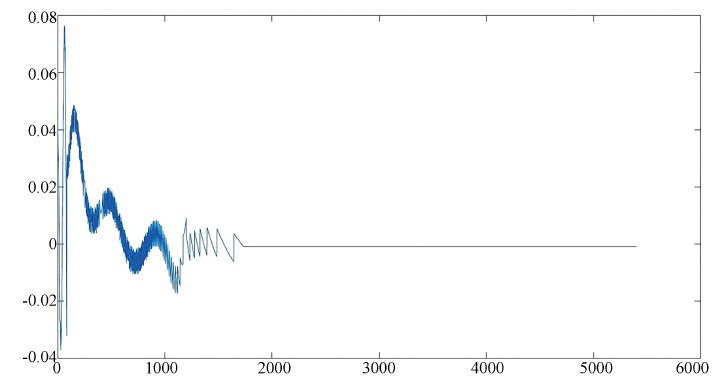Figure 3. Temperature time relationship of four layers of material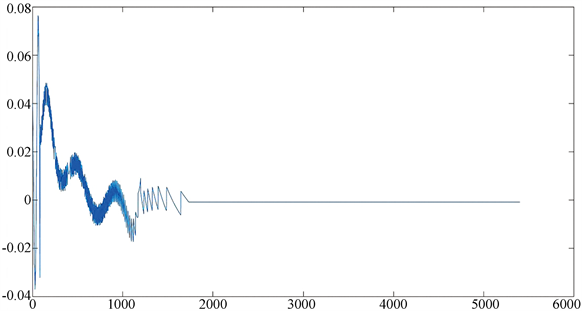Figure 4. Shows the difference between the data obtained and the given data

4.2. 问题二的分析、求解与检验

${d}_{2}$ 在微分方程中作为未知量，设法将 ${d}_{2}$ 作为一个新的变量添加到微分方程中，则此微分方程将多一个影响T的变量，这样就和偏微分方程一样，为了尽可能增加解题的可行性，提高计算精度，我们将 ${d}_{2}$ 作为t的函数即 ${d}_{2}\left(t\right)$ ，常数的导数为0可以作为一个微分方程添加到方程组中即 ${{d}^{\prime }}_{2}\left(t\right)=0$ ，如果这样将是一个可求的常微分方程组，将附件一与问题二的参数带入也可得到明确的微分方程组：

$\left\{\begin{array}{l}\frac{\text{d}{T}_{4}}{\text{d}t}=0.78052\left({T}_{3}-{T}_{4}\right)-0.78052\left({T}_{4}-37\right)\\ \frac{\text{d}{T}_{3}}{\text{d}t}=0.02712\left({T}_{2}-{T}_{3}\right)-\frac{\text{d}{T}_{4}}{\text{d}t}\\ \frac{\text{d}{T}_{2}}{\text{d}t}=2.04397×{10}^{-7}/\left({T}_{1}-{T}_{2}\right){d}_{2}^{2}\left(t\right)-\frac{\text{d}{T}_{3}}{\text{d}t}\\ {{d}^{\prime }}_{2}\left(t\right)=0\end{array}$ (式4.2.1)

$\left\{\begin{array}{l}{T}_{4}\left(3300\right)=44\\ {T}_{4}\left(3600\right)=47\\ {T}_{4}\left(0\right)=37\\ {T}_{3}\left(0\right)=37\\ {T}_{2}\left(0\right)=37\\ {T}_{1}\left(0\right)=37\end{array}$ (式4.2.2)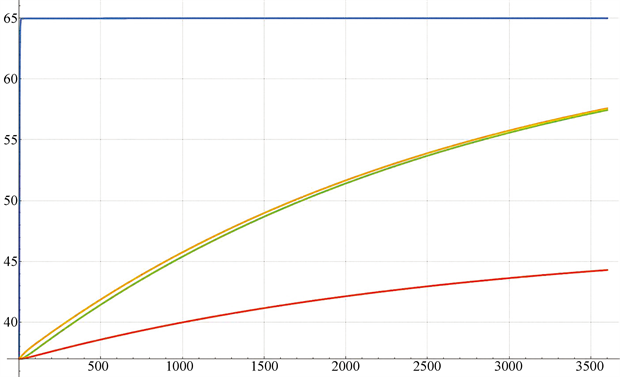Figure 5. Simulation image of four-layer material temperature increasing with time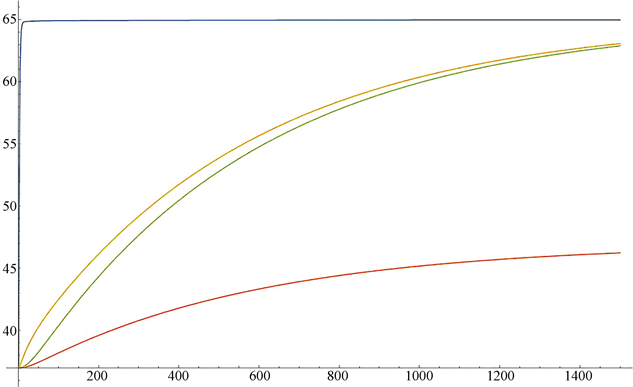Figure 6. Simulation image of four-layer material temperature increasing with time (1 s - 1600 s)

4.3. 问题三的分析、求解与检验

$\left\{\begin{array}{l}\frac{\text{d}{T}_{4}}{\text{d}t}=2.3611×{10}^{-5}\left({T}_{3}-{T}_{4}\right)/{d}_{4}^{2}\left(t\right)-2.3611×{10}^{-5}\left({T}_{4}-37\right)/{d}_{4}^{2}\left(t\right)\\ \frac{\text{d}{T}_{3}}{\text{d}t}=0.02712\left({T}_{2}-{T}_{3}\right)-\frac{\text{d}{T}_{4}}{\text{d}t}\\ \frac{\text{d}{T}_{2}}{\text{d}t}=2.04397×{10}^{-7}/{d}_{2}^{2}\left(t\right)\left({T}_{1}-{T}_{2}\right)-\frac{\text{d}{T}_{3}}{\text{d}t}\\ \frac{\text{d}{T}_{1}}{\text{d}t}=0.551386\left(65-{T}_{1}\right)-\frac{\text{d}{T}_{2}}{\text{d}t}\\ {{d}^{\prime }}_{2}\left(t\right)=0\\ {{d}^{\prime }}_{4}\left(t\right)=0\end{array}$ (式4.3.1)

$\left\{\begin{array}{l}{T}_{4}\left(1500\right)=44\\ {T}_{4}\left(1800\right)=47\\ {T}_{1}\left(0\right)=0\\ {T}_{2}\left(0\right)=0\\ {T}_{3}\left(0\right)=0\\ {T}_{4}\left(0\right)=0\\ {T}_{5}\left(0\right)=0\end{array}$ (式4.3.2)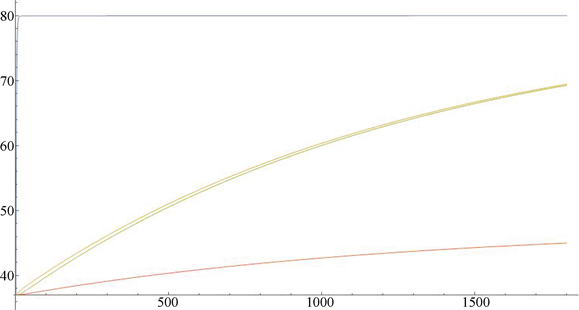Figure 7. Numerical solution (1 s - 1800 s)

5. 模型推广与改进

5.1. 实例应用

5.2. 模型改进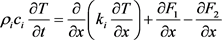( ${\rho }_{i}$ 为密度， ${c}_{i}$ 为对应比热， ${k}_{i}$ 为对应传导率， ${F}_{x}$ 为向左向右辐射量)

$\left\{\begin{array}{l}\frac{\partial {F}_{1}}{\partial x}=\beta {F}_{1}-\beta \sigma {T}^{4}\\ \frac{\partial {F}_{2}}{\partial x}=\beta {F}_{2}-\beta \sigma {T}^{4}\end{array}$ (式5.2.1)

$\beta$ 为辐射吸收常数单位； $\sigma$ 为斯蒂芬-波尔兹曼常数， 

$\left\{\begin{array}{l}c\rho \frac{\partial T}{\partial t}=\frac{\partial }{\partial x}\left(k\frac{\partial }{\partial x}\right)+\theta \left(x,t\right)\\ T\left(x,0\right)={T}_{1}\left(0\right)\\ -{k\frac{\partial T}{\partial t}|}_{x=0}={\left({q}_{conv}+{q}_{rad}\right)|}_{x=0}\end{array}$ (式5.2.2)

6. 模型评价

1) 对建立的高温作业服模型进行仿真的结果与附件一提供的现实数据相符合，说明了热传递模型的合理性。

2) 应用该模型预测仿真了织物层随时间的变化规律，便于观察分析。

3) 通过该模型可以确定每层的最优厚度，预测最长的安全工作时间，为热防护服的设计提供了科学理论依据。

4) 在进行模拟仿真的时候，将一维模型推广到三维模型，更符合实际情况。

5) 对模型进行了优化，由常微分计算后，在其基础上利用更加准确的偏微分进行了改进，与附件一所提供的数据基本一致，相互吻合，更加确切地说明了该模型的合理性。

6) 建立该模型时，详尽地考虑了各种可能性，在大量的物理原理基础上，使整个模型的构建更加符合现实世界，更具有科学性。

7) 采用计算机计算大量数据，并绘制大量模拟图，清晰明了，省时省力。

8) 可以看出改进模型考虑到了热传导在双向辐射下的影响，与物理现象更加贴切，并且经过资料查阅，我们在常微分方程的基础上采用偏微分方程，使整个模型更加趋于精准，计算主要利用Comsol软件的有限元分析模块，采用大量数据进行计算，与实况模型更加符合。

2018年全国大学生数学建模竞赛A题 附件一数见：

http://www.mcm.edu.cn/html_cn/node/7cec7725b9a0ea07b4dfd175e8042c33.html

 卢琳珍, 徐定华, 徐映红. 应用三层热防护服热传递改进模型的皮肤烧伤度预测[J]. 纺织学报, 2018, 39(1): 111-119.

 巩彦如, 张璇, 毛志平, 王新厚. 热防护纺织材料隔热性能的数值模拟[J]. 东华大学学报(自然科学版), 2010, 36(2): 115-117.

Top